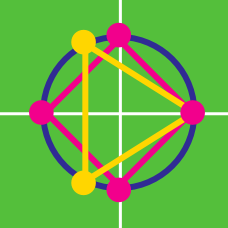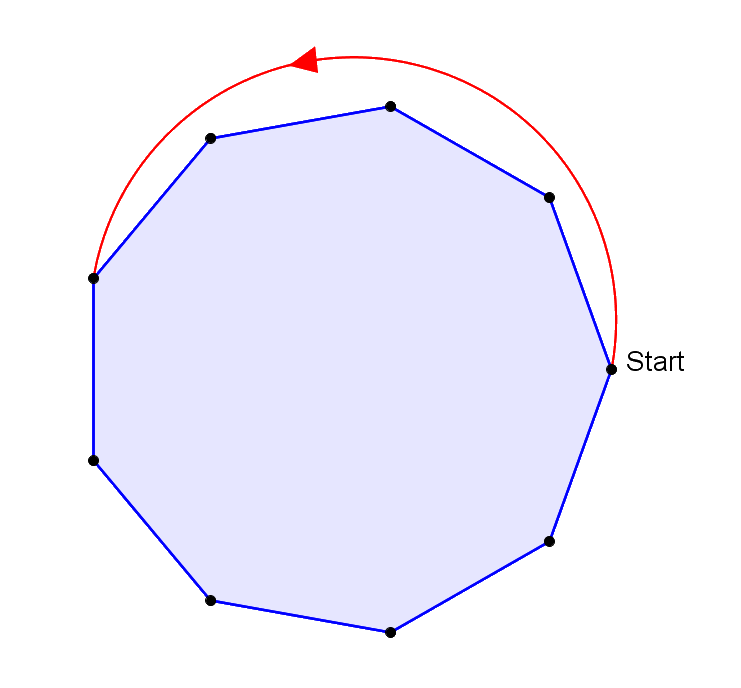Algebra

# Roots of Unity GeometryA "hop" is a movement of 4 sides at a time counterclockwise around the regular nonagon above. From the starting point, What is the minimum numbers of "hops" it will take to end up back at the starting point?

A regular hexagon has a vertex at $(1,0)$ and has its centroid at the origin. Which of these is another coordinate of the hexagon?

Regular polygons are placed on the coordinate plane such that they each have a vertex at $(1,0)$, and centroid at the origin. There is one regular $n$-gon for each value of $n$ between $3$ and $50$, inclusive.

How many values of $n$ are there such that the $n$-gon does not share any vertex other than $(1,0)$ with any of the other $n$-gons?

The point $(5,-3)$ is rotated $\dfrac{\pi}{4}$ radians counterclockwise about the point $(2,4)$. What is the resulting image?

Each of the partial sums $\sum\limits_{k=1}^{n}{e^{k\pi i/3}}$ is graphed on the complex plane for $n\in\{1,2,3,4,5,6\}$.

Which of the $6^\text{th}$ roots of unity coincides with one of these partial sums?

×

Problem Loading...

Note Loading...

Set Loading...